Johnny L. Hopkinshopkins28160

15 min

# Exploratory Data Analysis

Data science has become a common practice in global and domestic enterprises. Daily, companies are applying models developed through statistical methodologies to sell products, reduce customer churn, and increase the profitability of products. Before we can build predictive models, we first must look at basic statistical analysis. This is the basis of exploratory data analysis. In exploratory data analysis, the main emphasis is the graphical analysis of data sets plus the basic measures of central tendency. The ideas were developed in the 1970s by John Tukey and were mainly used in psychological data analysis, though now they have been incorporated into data science and analytics. In this blog post, we take the Ames Housing data set and perform basic exploratory data analysis on the SalePrice variable, the key variable used to build predictive models for real estate prices.

The Ames Housing data set was developed by Dean DeCock and was used in his statistics classes as a real-world way for his students to deal with raw data. We are using NumPy, Pandas, Matplotlib, and Seaborn. All of these modules form the basis of predictive modeling in Python using data science methods. To ensure our data is ready for analysis, we will clean the data and take a representative sample of the data. We have settled on taking 100 random values to analyze the SalePrice data from the larger 2000+ value data set. In statistics, we often take random samples to facilitate data analysis and statistical inference. With the following screenshots, we will examine how this is done using Pandas as well as taking the mean, median, variance, and standard deviation of our sample data. The first thing after we import NumPy, Pandas, Matplotlib, and Seaborn is to import the Ames data set using Pandas `read_csv()` function:Next, we output the first and last ten values of our data set to ensure it has been successfully read into our code: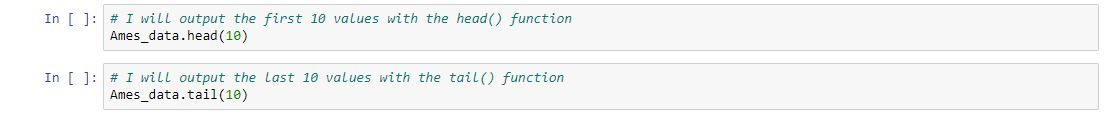Doing this results in this output: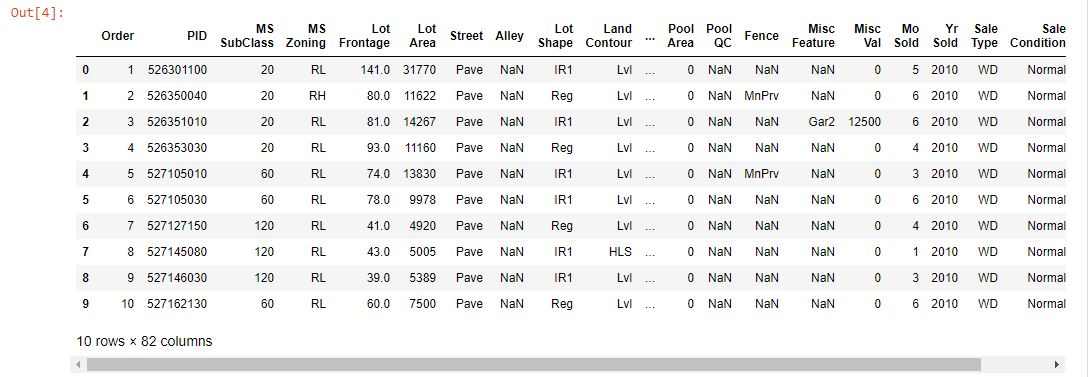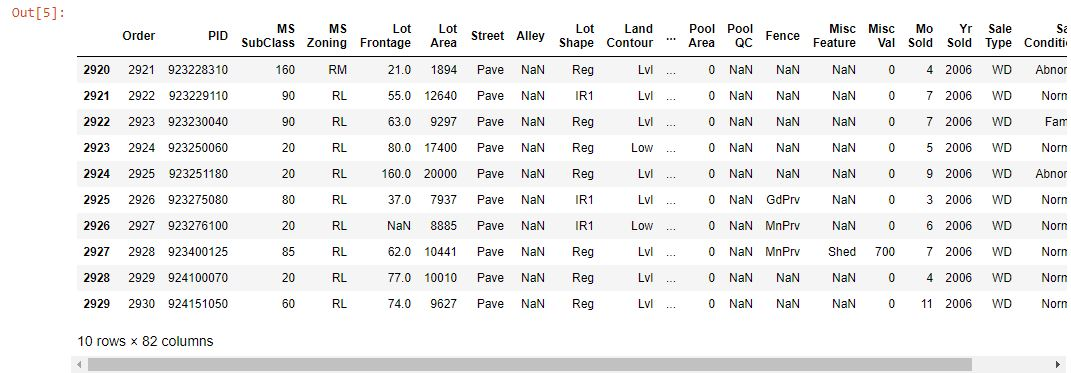Seeing the first ten and last ten values, we have missing values. These values have to be dropped using the `dropna()` method in Pandas. The `axis` parameter specifies the rows or columns where these missing values are found and deletes them. After taking a random 100 value sample with `sample()` and applying the `dropna()` function, we get the following result: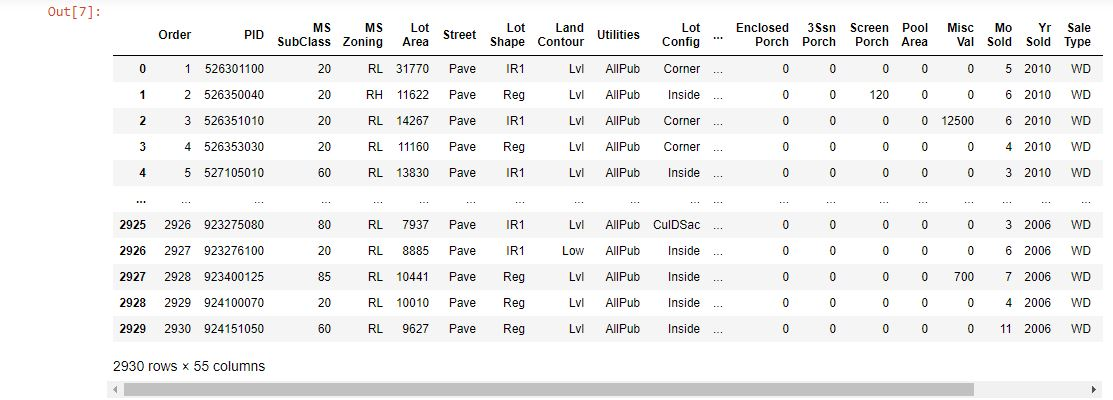Having cleaned our data set and picked our random values we apply the `mean()`, `median()`, `var()`, and `std()` methods to calculate our measures of central tendency. You can view and run the Jupyter notebook to see the values for yourself. To keep this blog post as brief as possible, I will show the result of plotting the distribution of sale prices using Seaborn. Seaborn is an alternative visualization library in Python which amplifies Matplotlib and gives the data scientist increased methods of plotting and analyzing data. Here is the graph: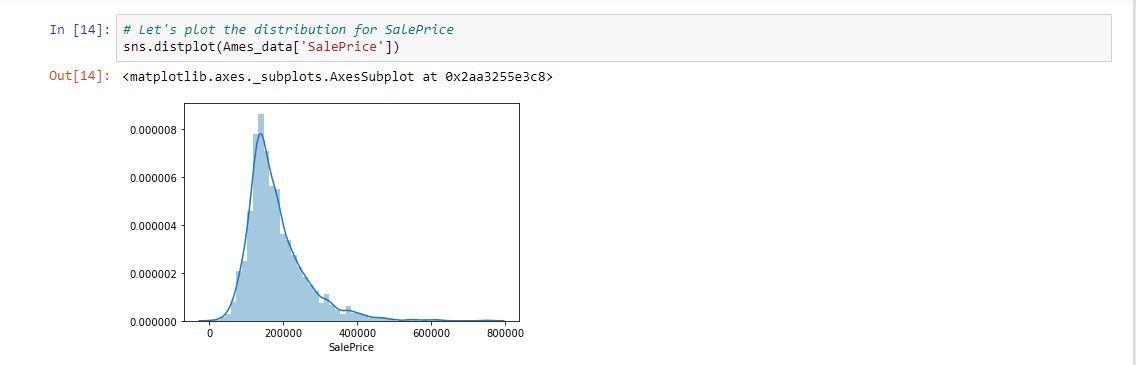The distribution of sales prices is skewed positively to the right, meaning that the mean > median. The graph indicates our sale prices in Ames, Iowa hover between \$100,000 and \$200,000 based on the curve. This is important if a homeowner wants to maximize their profit in selling their home. Using matplotlib, we could just plot the histogram like so: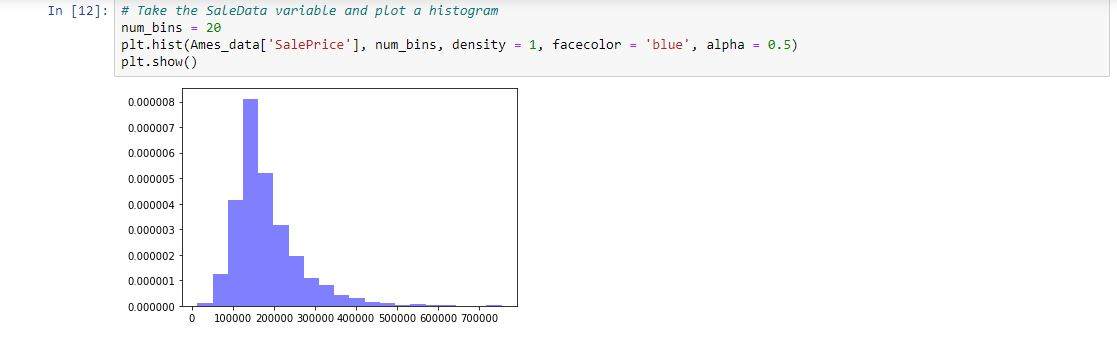So, our mean and median values for SalePrice hover around the aforementioned range.

In this post, we gave an example of exploratory data analysis of the Ames Housing data set using Python. We focused on SalePrice, but removed missing values and took a sample to facilitate data analysis. We looked at the measures of central tendency and plots to determine how the distribution of sale prices varied across price points. The reader is invited to follow this link and work with the notebook. In our next post, we will look at probability distributions using SciPy and graphically displaying them.